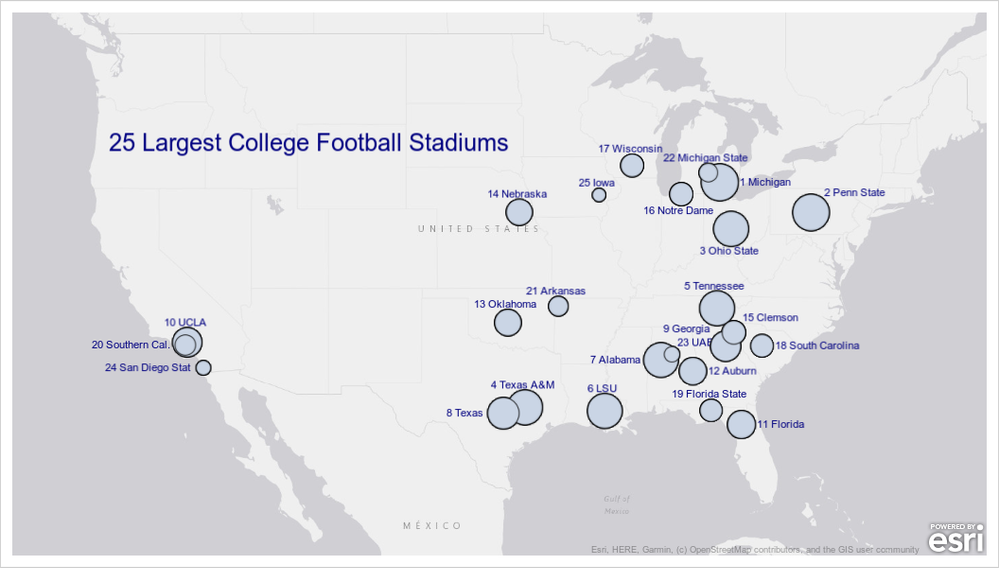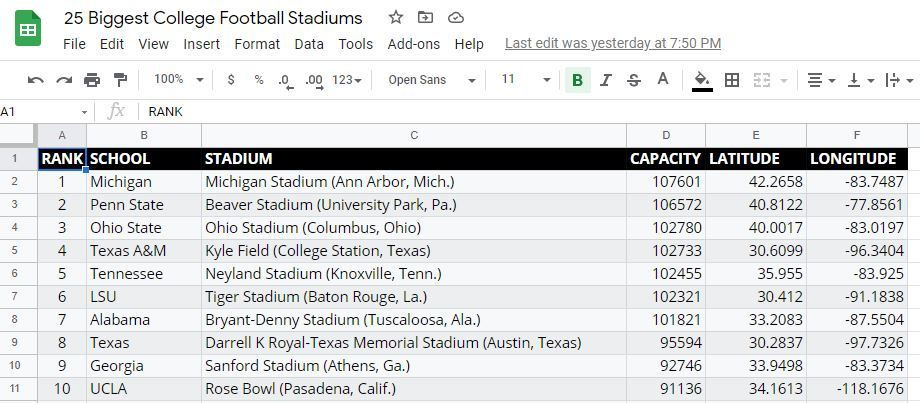## Fun With SAS ODS Graphics: The 25 Largest College Football StadiumsAre You Ready For Some Football?

After checking out the How Do I Show Geographical Data Using the SGMAP Procedure? on-demand seminar from @DanH_sas, how could I not try to create a map of the 25 biggest college football stadiums in the country? Btw, documentation shows there are LOTS more useful SGMAP BUBBLE statement options once I get access to SAS Viya 3.5, Post-9.4M6.

``````*  Fun With SAS ODS Graphics: The 25 Largest College Football Stadiums

data stadiums2; * Nudge Rank+Sachool (Rank+School) a bit to avoid collisions (Top/Middle/Bottom, Left/Center/Right);
select (school);
when ('San Diego State', 'Auburn', 'Michigan', 'South Carolina', 'Florida') schoolR=' '||catx(' ',rank,school);
when ('Southern Cal.', 'San Diego Stat', 'Texas', 'Alabama') schoolL=catx(' ',rank,school);
when ('Georgia') schoolTL=catx(' ',rank,school);
when ('Notre Damex') schoolBL=catx(' ',rank,school);
when ('Ohio State', 'Notre Dame') schoolB=catx(' ',rank,school);
when ('Clemson','UAB','Penn State') schoolTR=catx(' ',rank,school);
otherwise schoolT=catx(' ',rank,school);
end;

data chartdata; * Merge point data + heading;

ods graphics / reset height=6.25in width=11in antialias;
proc sgmap plotdata=chartdata noautolegend; * Chart data;
esrimap url='http://services.arcgisonline.com/arcgis/rest/services/Canvas/World_Light_Gray_Base';
bubble x=longitude y=latitude size=capacity / datalabel=schoolT  datalabelattrs=(size=8pt color=navy) datalabelpos=top; * Stadium points;
bubble x=longitude y=latitude size=capacity / datalabel=schoolR  datalabelattrs=(size=8pt color=navy) datalabelpos=right;
bubble x=longitude y=latitude size=capacity / datalabel=schoolL  datalabelattrs=(size=8pt color=navy) datalabelpos=left;
bubble x=longitude y=latitude size=capacity / datalabel=schoolTR datalabelattrs=(size=8pt color=navy) datalabelpos=topright;
bubble x=longitude y=latitude size=capacity / datalabel=schoolTL datalabelattrs=(size=8pt color=navy) datalabelpos=topleft;
bubble x=longitude y=latitude size=capacity / datalabel=schoolBL datalabelattrs=(size=8pt color=navy) datalabelpos=bottomleft;
bubble x=longitude y=latitude size=capacity / datalabel=schoolB  datalabelattrs=(size=8pt color=navy) datalabelpos=bottom;
run;``````

Sample Data (Full Data at Google Sheets)Sample Data

## Re: Fun With SAS ODS Graphics: The 25 Largest College Football Stadiums

I think you don't need separate variables for the labels, nor separate BUBBLE statements. You can put all labels in one variable:

``````data Have;
length Label \$10;
input x y size Label;
datalines;
0 0 102 Texas
0 3  86 Oklahoma
1 0 102 LSU
1 6  69 Iowa
2 8  80 Wisconson
3 2 101 Alabama
;

proc sgplot data=Have;
bubble x=x y=y size=size / datalabel=Label  datalabelattrs=(size=8pt color=navy) datalabelpos=top;
run;
``````
Discussion stats